# The Magnetic Field

When we began to study electric forces we introduced the concept of the electric field,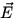. In particular, we had the fundamental relation between the force on a charge q in an electric field: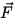= q. We will introduce a magnetic field in a similar manner. Before doing this, however, we define what is known as the cross productxbetween two vectorsand. Consider two such vectors in the plane of this page with an anglebetween them, as in Fig. 1.1.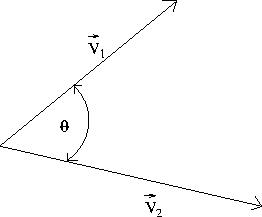x, which itself is a vector, is defined as follows:

• the magnitude ofxis given by

 |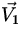x| = ||| sin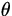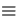V1V2sin, (1)

where ||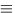V denotes the magnitude of a vector.
•xis a vector perpendicular to bothandwhose direction is given by the right-hand rule:
Point the thumb of your right hand in the direction ofand your fingers in the direction of. The direction ofxthen is directed out of the palm of your hand.
As the cross product of two vectors is three dimensional in nature, it is convenient to introduce the following notation for use on our two dimensional paper. We represent a vector perpendicular to the plane of this page which is directed out of the page as a circle with a black dot in the center - this can be thought of as the tip of an arrow coming towards you. On the other hand, a vector perpendicular to the plane of this page which is directed into the page is represented as a circle with a cross through the center - this can be thought of as the tail of an arrow heading away from you. Examples of this are in Fig. 1.2.We note two general features of the cross product:

•x= -•x= 0 ifandare parallel or antiparallel, as in these cases the anglebetween the two vectors is either 0 o or 180 o , for which the magnitude ofxgiven by Eq.(1.1) vanishes.
We now consider a charge q moving at velocity. If this charge is in a magnetic field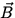, then it experiences a force given by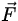= q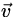x. (2)

From this we see that the units ofare Ns/(mC), which are given the special name Tesla (T). We note that, analogous to the electric field, for a given velocityand a given magnetic fieldthe force on a positive charge is in the opposite direction to the force on a negative charge.Next: Motion of a Charged Up: Magnetism Previous: Magnetism